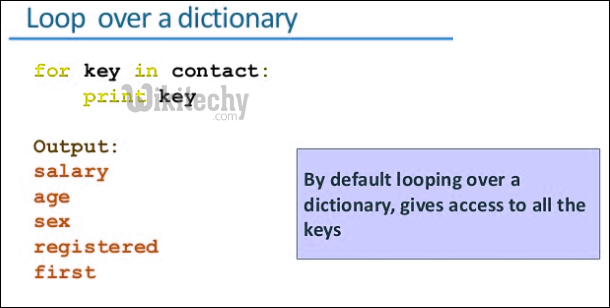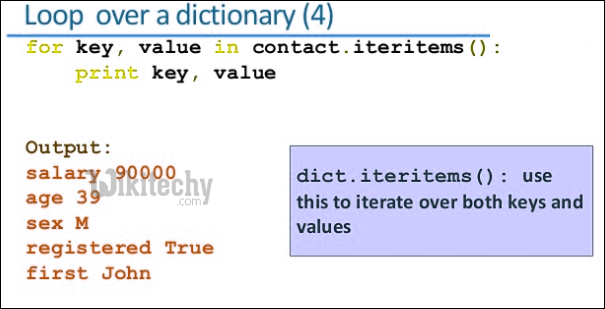# python tutorial - Python Dictionary Methods | Get() method for dictionaries in Python - learn python - python programmingLearn Python - Python tutorial - python dictionary getmethod - Python examples - Python programs

In python dictionaries, following is a conventional method to access a value for a key.python - Sample - python code :The problem that arises here is that the 3rd line of the code returns a key error :

python tutorial - Output :

```Traceback (most recent call last):
File ".\dic.py", line 3, in
print (dic["C"])
KeyError: 'C'
```The get() method is used to avoid such situations. This method returns the value for the given key, if present in the dictionary. If not, then it will return None (if get() is used with only one argument).

Syntax :

`Dict.get(key, default=None)`

Example:python - Sample - python code :

python tutorial - Output :

```1
None